# Texas Go Math Grade 2 Unit 3 Answer Key Algebraic Reasoning

Refer to our Texas Go Math Grade 2 Answer Key Pdf to score good marks in the exams. Test yourself by practicing the problems from Texas Go Math Grade 2 Unit 3 Answer Key Algebraic Reasoning.

## Texas Go Math Grade 2 Unit 3 Answer Key Algebraic Reasoning

Show What You Know

Check your understanding of important skills

Subtract Tens

Write the difference.

Question 1.5 tens – 3 tens = _________ tens
50 – 30 = _________
5 tens – 3 tens = 2 tens
50 – 30 = 20

Question 2.7 tens – 2 tens = _________ tens
70 – 20 = _________
7 tens – 2 tens = 5 tens
70 – 20 = 50

Write the sum.

Question 3.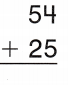Explanation:
The sum of 54 and 25 is 79.

Question 4.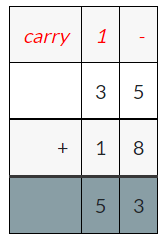Explanation:
The sum of 35 and 18 is 53.

Question 5.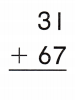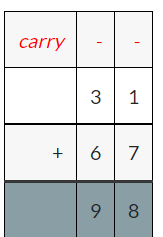Explanation:
The sum of 31 and 67 is 98.

Question 6.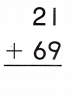Explanation:
The sum of 21 and 69 is 90.

Count by Tens

Question 7.
Count by tens. Write how many in all.__________ paints in all.
50 paints in all.

Explanation:
I counted by tens, there are 5 tens of paints in the image
5 tens is 50
Therefore, there are 50 paints in all.

FAMILY NOTE: This page checks your child’s understanding b of important skills needed for success in Unit 3.

Vocabulary Builder

Visualize it

Fill in the boxes of the graphic organizer. Write sentences using less and more.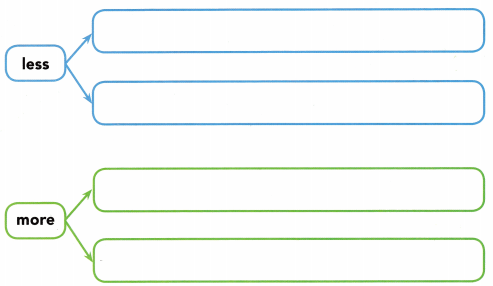Understand Vocabulary

Use the review words. Complete the sentences.

Review Words
less
more
digits
hundreds
tens

Question 1.
3 and 9 are ___________________ in the number 39.
3 and 9 are digits in the number 39.

Question 2.
8 is in the ____________________ place ¡n the number 87.
8 is in the tens place ¡n the number 87.

Question 3.
4 is in the ____________________ place ¡n the number 416.
4 is in the hundreds place ¡n the number 416.

You Can Be a Winner!
written by Tim Johnson illustrated by Lance Lekander

This Take-Home Book belongs to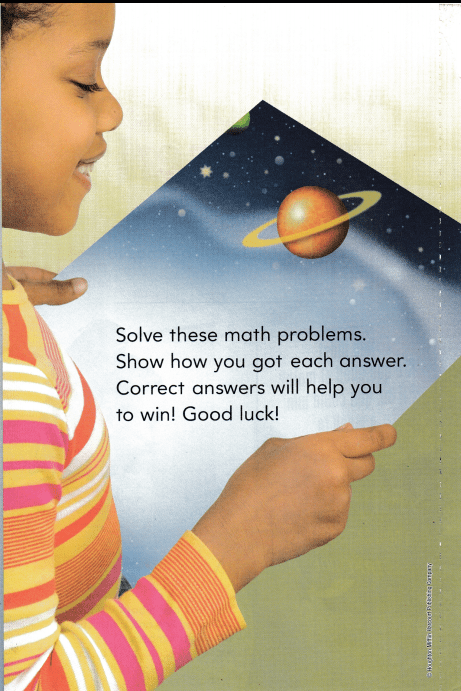Explanation:
94+97=191
Therefore, 191 children attended the space city camp in all.It takes Uranus about 84 years to orbit the sun.
It takes Saturn about 29 years to orbit the sun.
What is the difference in years?
________ – ___________ = __________ years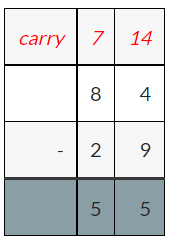Explanation:
84 – 29 = 55
The difference in the years of the planets to orbit the sun is 55 years.

47 small meteors hit the moon’s surface ¡n one hour.
65 hit the moon’s surface during the next hour.
How many meteors hit the moon’s surface in those two hours?
_____ + _____ = _____ meteorsExplanation:
47+65=112
112 meteors hit the moon’s surface in two hours.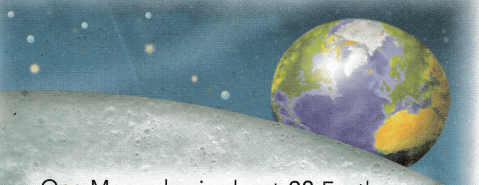One Moon day is about 28 Earth days long. How many Earth days are the same as 2 Moon days?
_____ + _____ = _____ Earth days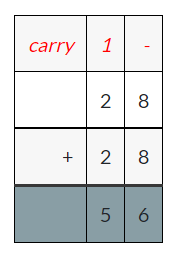Explanation:
28+28=56
56 days are the same as the 2 moon days.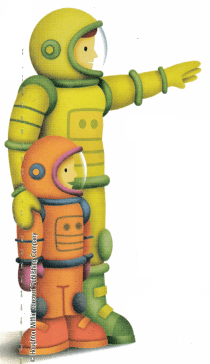Lucy is 8 years old now. She becomes an astronaut when she ¡s 35 years old. How many years until she becomes an astronaut?
_________ – ________ = _________ yearsExplanation:
35 – 8 = 27
There are 27 years until she became an astronaut.What do you think will happen? Write a few sentences to tell how this story will end.

Write Math Look at the picture of meteors hitting the moon. Write a story about the number of meteors hitting the moon on 2 different days. Use 2-digit numbers in your story.47 small meteors hit the moon’s surface on one day
65 hit the moon’s surface on the second day
How many meteors hit the moon’s surface on those two days?
_____ + _____ = _____ meteors

Use the information to answer the questions.

The Space Ride opens at 9:00 in the morning. In the first hour, 58 children go on the ride. In the next hour, 21 children go on the ride.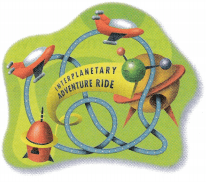Question 1.
How many children go on the ride in all?
________ children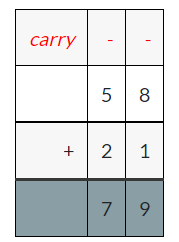Explanation:
58 + 21 = 79
Therefore, 79 children went to ride in all.

Question 2.
How many more children go on the Space Ride during the first hour than during the second hour?
_________ more children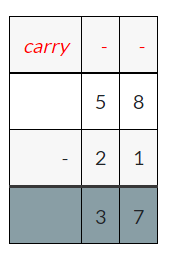Explanation:
58 – 21 = 37
Therefore, 37 more children went on the Space Ride during the first hour than during the second hour.

Question 3.
In the third hour, 37 children ride on the Space Ride. How many children go on the ride in the first 3 hours that it is open?
________ childrenExplanation:
58+21+37=116
Therefore, 116 children went on the Space Ride. How many children go on the ride in the first 3 hours that it is open.

MATH BOARD

Write a problem about the Space Ride that uses 2-digit addition or subtraction. Have a classmate solve the problem. Talk about strategies you can use to solve the problem.